Maximum Possible Edge Disjoint Spanning Tree From a Complete Graph

• Last Updated : 14 Apr, 2021

Give a complete graph with N-vertices. The task is to find out the maximum number of edge-disjoint spanning tree possible.
Edge-disjoint Spanning Tree is a spanning tree where no two trees in the set have an edge in common.
Examples:

Input : N = 4
Output : 2

Input : N = 5
Output : 2

The maximum number of possible Edge-Disjoint Spanning tree from a complete graph with N vertices can be given as,

Max Edge-disjoint spanning tree = floor(N / 2)

Let’s look at some examples:
Example 1:

Complete graph with 4 vertices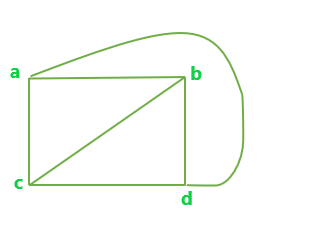All possible Edge-disjoint spanning trees for the above graph are: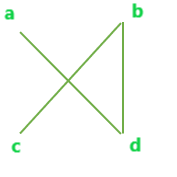A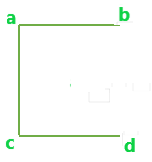B

Example 2

Complete graph with 5 vertices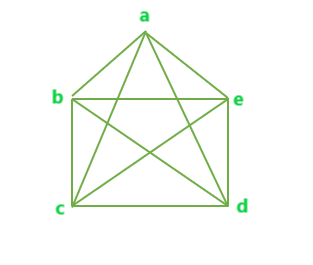All possible Edge-disjoint spanning trees for the above graph are: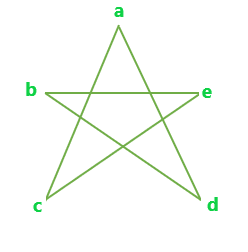A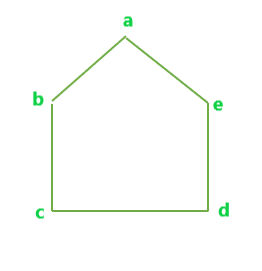B

Below is the program to find the maximum number of edge-disjoint spanning trees possible.

C++

 // C++ program to find the maximum number of // Edge-Disjoint Spanning tree possible   #include using namespace std;   // Function to calculate max number of // Edge-Disjoint Spanning tree possible float edgeDisjoint(int n) {     float result = 0;       result = floor(n / 2);       return result; }   // Driver code int main() {     int n = 4;       cout << edgeDisjoint(n);       return 0; }

Java

 // Java program to find the maximum  // number of Edge-Disjoint Spanning // tree possible import java.io.*;   class GFG {       // Function to calculate max number // of Edge-Disjoint Spanning tree // possible static double edgeDisjoint(int n) {     double result = 0;       result = Math.floor(n / 2);       return result; }   // Driver Code public static void main(String[] args) {     int n = 4;     System.out.println((int)edgeDisjoint(n)); } }   // This code is contributed // by Naman_Garg

Python3

 # Python 3 to find the maximum # number of Edge-Disjoint # Spanning tree possible import math   # Function to calculate max # number of Edge-Disjoint # Spanning tree possible def edgeDisjoint(n):       result = 0       result = math.floor(n / 2)       return result   # Driver Code if __name__ == "__main__" :       n = 4       print(int(edgeDisjoint(n)))   # This Code is contributed # by Naman_Garg

C#

 // C# program to find the maximum number of // Edge-Disjoint Spanning tree possible using System;   class GFG {   // Function to calculate max number of // Edge-Disjoint Spanning tree possible static double edgeDisjoint(double n) {     double result = 0;       result = Math.Floor(n / 2);       return result; }   // Driver Code public static void Main() {     int n = 4;       Console.Write(edgeDisjoint(n)); } }   // This code is contributed // by Sanjit_Prasad



Javascript



Output:

2

My Personal Notes arrow_drop_up
Recommended Articles
Page :